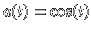Inverted pendulum

 A pendulum with vertically oscillating point of suspension is an example of a pendulum with periodic forceLinearizing around an equilibrium pointgives the Mathieu type equationwhere a(t) is a periodic function. Ifand, this linear differential equation Lx=0 is called the Mathieu equation. The differential operator L is called the Mathieu operator. For fixed, the set of parameters g for which x=0 is stable form form a union of intervals called stable bands. The complement is a set of unstable bands. The union of all unstable bandsis a union of Arnold tongues. If a(t) oscillates fast enough, the pointcan become stable. In the case of a piecewise constantfor example, a pendulum of length 20cm with suspension oscillation 1cm becomes stable at 43 Hertz (see V. Arnold, Mathematical Methods of classical mechanics p. 122).

 © 2000, Oliver Knill , dynamical-systems.org